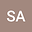Aronson-B\’{e}nilan estimates for weighted porous medium equations under the geometric flow
•Abstract

In this paper, we study Aronson-B\’{e}nilan gradient estimates for positive solutions of weighted porous medium equations $$\partial_{t}u(x,t)=\Delta_{\phi}u^{p}(x,t),\,\,\,\,(x,t)\in M\times[0,T]$$ coupled with the geometric flow $\frac{\partial g}{\partial t}=2h(t),\,\,\,\frac{\partial \phi}{\partial t}=\Delta \phi$ on a complete measure space $(M^{n},g,e^{-\phi}dv)$. As an application, by integrating the gradient estimates, we derive the corresponding Harnack inequalities.

Peer review status:UNDER REVIEW

21 Nov 2021Submitted to Mathematical Methods in the Applied Sciences
23 Nov 2021Assigned to Editor
23 Nov 2021Submission Checks Completed
28 Nov 2021Reviewer(s) Assigned